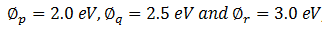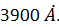Courses

# Dual Nature Of Matter And Radiation - Online Test (05-01-2017)

## 30 Questions MCQ Test | Dual Nature Of Matter And Radiation - Online Test (05-01-2017)

Description
This mock test of Dual Nature Of Matter And Radiation - Online Test (05-01-2017) for Class 12 helps you for every Class 12 entrance exam. This contains 30 Multiple Choice Questions for Class 12 Dual Nature Of Matter And Radiation - Online Test (05-01-2017) (mcq) to study with solutions a complete question bank. The solved questions answers in this Dual Nature Of Matter And Radiation - Online Test (05-01-2017) quiz give you a good mix of easy questions and tough questions. Class 12 students definitely take this Dual Nature Of Matter And Radiation - Online Test (05-01-2017) exercise for a better result in the exam. You can find other Dual Nature Of Matter And Radiation - Online Test (05-01-2017) extra questions, long questions & short questions for Class 12 on EduRev as well by searching above.
QUESTION: 1

Solution:
QUESTION: 2

Solution:
QUESTION: 3

### As per Bohr model, the minimum energy (in eV) required to remove an electron from the ground state of double ionzed Li atom (Z = 3) is:

Solution:
QUESTION: 4

A proton, a deutron and an alpha particle are accelerated through potentials of V, 2V and 4V respectively. Their velocities will bear a ratio.

Solution:
QUESTION: 5

The work function of a substance is 4.0 eV. The longest wavelength of light that can cause photoelectron emission from this substance is approximately

Solution:
QUESTION: 6

An electron is 2000 times lighter than a proton. Both are moving such that their matter waves have a length of 1Å. The ratio of their kinetic energy in approximation is

Solution:
QUESTION: 7

The Ka  X-ray emission line of tungsten occurs at λ = 0.021 nm. The energy difference between K and L levels in this atom is about

Solution:
QUESTION: 8

The maximum kinetic energy of photoelectrons emitted from a surface when photons of energy 6eV fall on it is 2eV. The stopping potential in volts is:

Solution:
QUESTION: 9

An electron enters electric field of 104 B/m perpendicular to the field with a velocity of 107 m/s. The vertical displacement of electron after one mille second will be:

Solution:
QUESTION: 10

Half lives of two radioactive elements A and B are 20 min and 40 min respectively. Initially the samples have equal number of nuclei. After 80 min the ratio of decayed numbers of A and B is

Solution:
QUESTION: 11

As an electron makes a transition from an excited state to ground state of hydrogen like atom/ion

Solution:
QUESTION: 12

The radiation corresponding 3  →  2 transition of a hydrogen atom falls on a metal surface to produce photoelectrons. These electrons are made to enter in the magnetic field of 3 X 10-4T. if the radius of the largest circular path followed by these electrons is 10.0 mm, the work function of the metal is close to

Solution:
QUESTION: 13

A radiation of energy E falls normally on a perfectly reflecting surface. The momentum  transferred to the surface is (velocity of light = c)

Solution:
QUESTION: 14

A source of light is placed at a distance of 1 m from a photocell and cut-off potential is found to be V0 . if the distance is doubled, the cut-off potential will be:

Solution:
QUESTION: 15

When 1 centimetre thick surface is illuminated with light of wavelength λ, the stopping potential is V. when the same surface is illuminated by light of wavelength 2 λ, the stopping potential is V/3. Threshold wavelength for metallic surface is:

Solution:
QUESTION: 16

We wish to see inside an atom. Assuming the atom to have a diameter of 100 pm [ 1 picometer (pm) = 10-12 m], this means that one must be able to resolve a width of, say 10 pm. If an electron microscope is used, the minimum electron energy required is about :

Solution:
QUESTION: 17

An electron of mass m, when accelerated through a potential difference V, has de Broglie wavelength λ. The de Broglie wavelength associated with a proton of mass M accelerated through the same potential difference will be :

Solution:
QUESTION: 18

If particles are moving with same velocity, then which has maximum de Broglie wavelength?

Solution:
QUESTION: 19

The wavelength λe of an electron and λp of a  photon of the same energy E are related by :

Solution:
QUESTION: 20

Photoelectric effect experiments are performed using three different metal plates p, q and r having work functions, respectively. A light beam containing wavelengths of 550 nm, 450 nm and 350 nm with equal intensities illuminates each of the plates. The correct I-V graph for the experiment is :

[take hc =1240 eV nm]

Solution:
QUESTION: 21

A metal surface is illuminated by light of two different wavelengths, 248 nm and 310 nm. The maximum speeds of the photoelectrons corresponding to these wavelengths are μ1 and μ2  respectively. If the ratio  μ1 : μ2  = 2:1 and hc = 1240 eV nm, the work function of the metal is nearly :

Solution:
QUESTION: 22

Two identical photo cathodes receive lights of frequencies f1 and f2. If the velocity of the photoelectrons ( of mass m) coming out are respectively v1 and v2, then :

Solution:
QUESTION: 23

The anode voltage of a photocell is kept fixed. The wavelength λ of the light falling on the cathode in gradually changed. The plate current I of the photocell varies as follows :

Solution:
QUESTION: 24

An image of the sun is formed by a lens of focal length 30 cm on the metal surface of a photoelectric  cell and it produces a current I . The lens forming the image is then replaced by another lens of the same diameter but of focal length 15 cm. the photoelectric current in this case will be :

Solution:
QUESTION: 25

Shining light of wavelength λ and intensity I on a surface S produces photoelectrons at rate R and with maximum kinetic energy E. consider the following statements for the effect of changing one parameter at a time:

(i)  doubling I always doubles R

(ii) doubling I does not change E at all

(iii)  making λ half always makes E more than 2 fold.

The statements true are :

Solution:
QUESTION: 26

According to Einstein’s photoelectric equation, the graph between the kinetic energy of photoelectrons ejected and the frequency of incident radiation is :

Solution:
QUESTION: 27

The threshold wavelength for photoelectric emission form a material is. Photoelectrons will be emitted when this material is illuminated with monochromatic radiation from :

Solution:
QUESTION: 28

If λ0 stands for mid-wavelength in the visible region, the de Broglie wavelength for 100 V electrons is nearest to

Solution:
QUESTION: 29

Maximum velocity of the photoelectrons emitted by a metal surface is 1.2 × 106 m/s. assuming the specific charge of the electron to be 1.8 × 1011 C/kg, the value of the stopping potential in volt will be:

Solution:
QUESTION: 30

Light from a hydrogen discharge tube is incident on the cathode of a photoelectric cell. The work function of the cathode surface is 4.2 eV. In order to reduce the photocurrent to zero, the voltage of the anode relative to the cathode must be made:

Solution: